Author: Oscar Cronquist Article last updated on February 01, 2019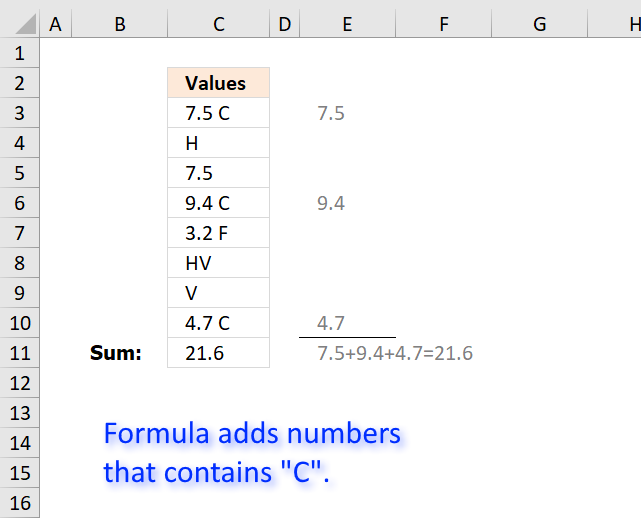Question:

I want to sum cells that have a "C" and a decimal number.

The cells have other numbers and variables in them as well, but I only want to add ones
with "C"s.

Example of what cells contain:
7.5 C
H
7.5
9.4 C
3.2 F
HV
V
4.7 C

The array formula in the picture above searches for string "C" in cell range C3:C10 and extracts the corresponding number part.

Array formula in cell C11:

=SUM(IF(ISNUMBER(SEARCH("C", C3:C10)), LEFT(C3:C10, LEN(C3:C10)-1)*1, ""))

To enter an array formula, type the formula in cell B3 then press and hold CTRL + SHIFT simultaneously, now press Enter once. Release all keys.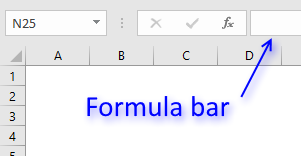The formula bar now shows the formula with a beginning and ending curly bracket telling you that you entered the formula successfully.

Don't enter the curly brackets yourself, they appear automatically.

### Explaining formula

Use "Evaluate Formula" on tab "Formula" on the ribbon to go through the steps in the formula.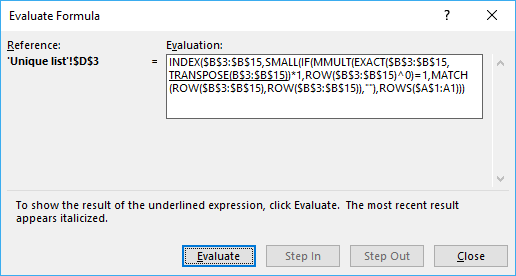#### Step 1 - Find values containing text string

The SEARCH function allows you to search for a specific string in a cell range or array.

becomes

SEARCH("C", {"7.5 C";"H";7.5;"9.4 C";"3.2 F";"HV";"V";"4.7 C"})

and returns {5; #VALUE!; #VALUE!; 5;#VALUE!; #VALUE!; #VALUE!; 5}.

The issue with this array is that the SEARCH function returns a #VALUE error if the string is not found.

Number 5 is where "C" is found in the string, in other words "C" is found in position 5 counting from the left.

For example, 7.5 C has five characters, "C" is the fifth character in the string.

#### Step 2 - Identify numbers in array

The ISNUMBER function handles error values in a great way, instead of returning the error value it converts it to FALSE.

becomes

ISNUMBER({5; #VALUE!; #VALUE!; 5;#VALUE!; #VALUE!; #VALUE!; 5})

and returns {TRUE; FALSE; FALSE; TRUE; FALSE; FALSE; FALSE; TRUE}.

We now have an array without error values that is easier to work with.

TRUE indicates that the corresponding cell contains the string "C" and FALSE that "C" is missing.

#### Step 3 - Remove C from value and convert it to a numerical value.

Since C is the last character we can use the LEFT function to extract a given number of characters.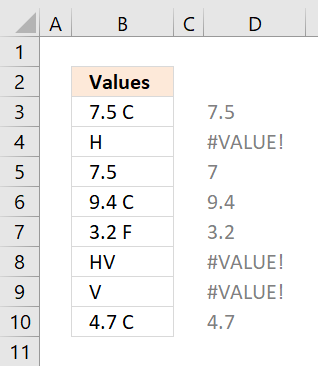The LEN function counts the number of characters in each cell. Subtracting with 1 returns how many characters we need to extract counting from left.

LEFT(C3:C10, LEN(C3:C10)-1)*1

becomes

LEFT(C3:C10, {4; 0; 2; 4; 4; 1; 0; 4})*1

becomes

{"7.5 ";"";"7.";"9.4 ";"3.2 ";"H";"";"4.7 "}*1

To convert text values to numerical values I multiply with 1.

{"7.5 "; ""; "7."; "9.4 "; "3.2 "; "H"; ""; "4.7 "}*1

becomes

{7.5; #VALUE!; 7; 9.4; 3.2; #VALUE!; #VALUE!; 4.7}

We can now use this array to replace the boolean array if value is TRUE.

#### Step 4 - Convert boolean values to corresponding numbers

The IF function allows you to use the boolean array and replace it with numbers (if TRUE) or nothing (if FALSE).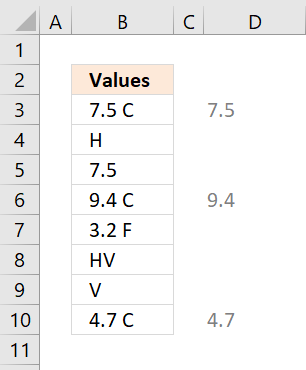IF(ISNUMBER(SEARCH("C", C3:C10)), LEFT(C3:C10, LEN(C3:C10)-1)*1, "")

becomes

IF({TRUE; FALSE; FALSE; TRUE; FALSE; FALSE; FALSE; TRUE}, {7.5; #VALUE!; 7; 9.4; 3.2; #VALUE!; #VALUE!; 4.7}, "")

and returns {7.5; ""; ""; 9.4; ""; ""; ""; 4.7}.

The error values are ignored and "" (nothing) is returned in those locations.

#### Step 5 - SUM numerical values ignoring blank values

The SUM function is intelligent, it ignores blank and text values.

SUM(IF(ISNUMBER(SEARCH("C", C3:C10)), LEFT(C3:C10, LEN(C3:C10)-1)*1, ""))

becomes

SUM({7.5; ""; ""; 9.4; ""; ""; ""; 4.7})

and returns 21.6 in cell C11.### Get the Excel fileSum-text-cells-using-criteria.xlsx